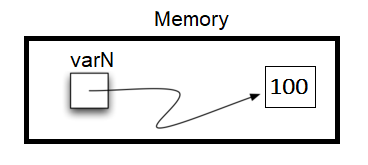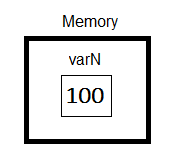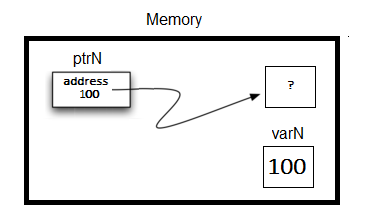# 2.1. Using Data in C++¶

C++ requires the users specify the specific data type of each variable before it is used. The primary C++ built-in atomic data types are: integer (int), floating point (float), double precision floating point (double), Boolean (bool), and character (char). There is also a special type which holds a memory location called pointer. C++ also has collection or compound data types, which will be discussed in a future chapter.

# 2.2. Numeric Data¶

Numeric C++ data types include int for integer, float for floating point, double for double precision floating point.

The standard arithmetic operations, +, -, *, and / are used with optional parentheses to force the order of operations away from normal operator precedence.

In Python we can use // to get integer division. In C++, we declare all data types. When two integers are divided in C++, the integer portion of the quotient is returned and the fractional portion is removed. i.e. When two integers are divided, integer division is used. To get the whole quotient, declaring one of the numbers as a float will convert the entire result into floating point.

Exponentiation in C++ is done using pow() from the cmath library and the remainder (modulo) operator is done with %.

Run the following code to see that you understand each result.

When declaring numeric variables in C++, modifiers like short, long, and unsigned can optionally be used to help to ensure space is used as efficiently as possible.

Q-1: what is the result of dividing 3/2 in C++?

• 1
• Right! This is like 3//2 in Python.
• 1.5
• No. Integer division is used. Try again.
• 2
• No. Integer division is used. Try again.
• A run-time error will occur.
• No, C++ generally will try to do as you ask.
• none of the above
• One of the above is correct.

Q-2: How do I raise 4 to 5th power in C++?

• 4**5
• No, ** is used in Python, not C++.
• 5**4
• No, ** is used in Python, not C++, and the operators are reversed.
• 4^5
• No. The ^ is a valid operator in C++, but it does something else.
• pow(4, 5)
• You got it! Remember the cmath library will need to be included for pow() to work.

# 2.3. Boolean Data¶

Boolean data types are named after George Boole who was an English mathematician, so the word “Boolean” should be capitalized. However, the Boolean data type, in C++ uses the keyword bool which is not capitalized. The possible state values for a C++ Boolean are lower case true and false. Be sure to note the difference in capitalization from Python. In Python, these same truth values are capitalized, while in C++, they are lower case.

C++ uses the standard Boolean operators, but they are represented differently than in Python: “and” is given by && , “or” is given by || , and “not” is given by !. Note that the internally stored values representing true and false are actually 1 and 0 respectively. Hence, we see this in output as well.

Boolean data objects are also used as results for comparison operators such as equality (==) and greater than ($$>$$). In addition, relational operators and logical operators can be combined together to form complex logical questions. Table 1 shows the relational and logical operators with examples shown in the session that follows.

Table 1: C++ Relational and Logical Operators

Operation Name

Operator

Explanation

less than

$$<$$

Less than operator

greater than

$$>$$

Greater than operator

less than or equal

$$<=$$

Less than or equal to operator

greater than or equal

$$>=$$

Greater than or equal to operator

equal

$$==$$

Equality operator

not equal

$$!=$$

Not equal operator

logical and

$$&&$$

Both operands true for result to be true

logical or

$$||$$

One or the other operand is true for the result to be true

logical not

$$!$$

Negates the truth value, false becomes true, true becomes false

When a C++ variable is declared, space in memory is set aside to hold this type of value. A C++ variable can optionally be initialized in the declaration by using a combination of a declaration and an assignment statement.

Consider the following session:

The declaration int theSum = 0; creates a variable called theSum and initializes it to hold the data value of 0. As in Python, the right-hand side of each assignment statement is evaluated and the resulting data value is “assigned” to the variable named on the left-hand side. Here the type of the variable is integer. Because Python is dynamically typed, if the type of the data changes in the program, so does the type of the variable. However, in C++, the data type cannot change. This is a characteristic of C++’s static typing. A variable can hold ever only one type of data. Pitfall: C++ will often simply try to do the assignment you requested without complaining. Note what happened in the code above in the final output.

Q-3: Why did theBool output a value of 1 after being set to 4?

• Setting theBool to anything other than true or false is ignored.
• No. Try changing the code and setting theBool to 0.
• Setting theBool to anything > 0 will be true and false otherwise.
• No. Try changing the code and setting theBool to -4.
• false == 0 and true = !false i.e. anything that is not zero and can be converted to a Boolean is not false, thus it must be true.
• Correct!
• None of the above.
• Try again. One of the above is correct. You might try changing the code and rerunning.

# 2.4. Character Data¶

In Python strings can be created with single or double quotes. In C++ single quotes are used for the character (char) data type, and double quotes are used for the string data type.

Consider the following code.

Try the following question.

Q-4: If I want to create a string in C++, what set of symbols may be used?

• ' '
• No, single quotes are only used for single characters.
• " "
• ' ' or " " may be used
• No. Only one set of symbols may be used.
• It depends upon the implementation.
• No. The implementation is consistent.
• none of the above
• One of the above is indeed correct.

# 2.5. Pointers¶

A C++ pointer is a variable that stores a memory address and can be used to indirectly access data stored at that memory location.

We know that variables in a computer program are used to label data with a descriptive identifier so that the data can be accessed and used by that computer program.

Let’s look at some examples of storing an integer in Python and C++.

In Python every single thing is stored as an object. Hence, a Python variable is actually a reference to an object that is stored in memory. Hence, each Python variable requires two memory locations: one to store the reference, and the other to store the variable value itself in an object.

In C++ the value of each variable is stored directly in memory without the need for either a reference or an object. This makes access faster, but it is one of the reasons we need to declare each variable because different types take differing amounts of space in memory!

The following code declares a variable called varN that has in it a value of 100:

// Python reference for a single integer value
varN = 100Figure 2.1: Python reference

// C++ variable declaration and assignment of an integer value
int varN = 100;


In C++ the results of running this code will look like the diagram below:Figure 2.2: C++ variable

In each case, when we want to output the value to the console, we use the variable name to do so.

But, we can also identify the memory location of the variable by its address. In both Python and C++, this address may change each time the program is run. In C++, the address will always look odd because it will be the actual memory address written in a hexadecimal code which is a base 16 code like 0x7ffd93f25244. In Python it is implementation dependent, it is sometimes a hexadecimal code and sometimes just a count or another way to reference the address.

In Python we use id to reference the address, while in C++ we use the address-of operator, &.

In both Python and C++, variables are stored in memory locations which are dependent upon the run itself. If you repeatedly run the above code in either C++ or Python, you may see the location change.

As suggested above, in Python, it is impossible to store a variable directly. Instead, we must use a variable name and a reference to the data object. (Hence the arrow in the image.) In C++, variables store values directly, because they are faster to reference.

References are slower, but they are sometimes useful. If in C++, we want to create a analogous reference to a memory location, we must use a special data type called a pointer.

## 2.5.1. Pointer Syntax¶

When declaring a pointer in C++ that will “point” to the memory address of some data type, you will use the same rules of declaring variables and data types. The key difference is that there must be an asterisk (*) between the data type and the identifier.

variableType *identifier; // syntax to declare a C++ pointer
int *ptrx; // example of a C++ pointer to an integer


White space in C++ generally does not matter, so the following pointer declarations are identical:

SOMETYPE *variablename; // preferable
SOMETYPE * variablename;
SOMETYPE* variablename;


However, the first declaration is preferable because it is clearer to the programmer that the variable is in fact a pointer because the asterisk is closer to the variable name.

### 2.5.1.1. The address-of operator, &¶

Now that we know how to declare pointers, how do we give them the address of where the value is going to be stored? One way to do this is to have a pointer refer to another variable by using the address-of operator, which is denoted by the ampersand symbol, &. The address-of operator & does exactly what it indicates, variableType varN; // a variable to hold the value namely it returns the address.

The syntax is shown below, where varN stores the value, and ptrN stores the address of where varN is located:

variableType *ptrN = &varN;  // a variable pointing to the address of varN


Keep in mind that when declaring a C++ pointer, the pointer needs to reference the same type as the variable or constant to which it points.

Expanding on the example above where varN has the value of 9.

//variable declaration for a single integer value
int varN = 9;
int *ptrN;
ptrN = &varN;


The results of running this C++ code will look like the diagram below.Figure 2.3: View into memory with pointers

## 2.5.2. Accessing Values from Pointers¶

Once you have a C++ pointer, you use the asterisk before the pointer variable, to dereference the pointer, which means go to the location pointed at by the 3.

In other words, varN and *ptrN (note the asterisk in front!) reference the same
value in the code above.


Let’s extend the example above to output the value of a variable and its address in memory:

Q-5: If the lines (varN = 50;) and (cout << *ptrN << endl;) were inserted into line 7-8, what would it cout?

• varPntr: 9
• Not quite, the variable varN no longer equals 100 past line 7!
• varPntr: 50
• Right!
• varPntr: 150
• No, the values do not add together!
• 0x7ffeb9ce053c
• We are dereferencing the pointer, so you would not get the address of varN. Try again!
• none of the above
• One of the above is indeed correct.

Compiling and running the above code will have the program output the value in varN, what is in ptrN (the memory address of varN), and what value is located at that memory location.

The second output sentence is the address of varN, which would most likely be different if you run the program on your machine.

WARNING: What happens if you forget the asterisk when assigning a value to a pointer and had the following instructions instead?Figure 2.4: dangling pointer reference

If your compiler does not catch that error (the one for this class may), the first cout instruction outputs

After changing *ptrN, varN now has: 50


which is expected because you changed where ptrN is pointing to and NOT the contents of where it is pointing.

The second cout instruction is a disaster because (1) You don’t know what is stored in location 100 in memory, and (2) that location is outside of your segment (area in memory reserved for your program), so the operating system will jump in with a message about a “segmentation fault”. Although such an error message looks bad, a “seg fault” is in fact a helpful error because unlike the elusive logical errors, the reason is fairly localized.

## 2.5.3. The null pointer¶

Like None in Python, the null pointer (nullptr) in C++ points to nothing. Older editions of C++ also used NULL (all caps) or 0, but we will use the keyword nullptr because the compiler can do better error handling with the keyword. The null pointer is often used in conditions and/or in logical operations.

The following example demonstrates how the null pointer works. The variable ptrx initially has the address of x when it is declared. On the first iteration of the loop, it is assigned the value of nullptr, which evaluates to a false value; thereby ending the loop:

Helpful Tip: The null pointer becomes very useful when you must test the state of a pointer, such as whether the assignment to an address is valid or not.

# 2.6. Summary¶

1. All variables must be declared before use in C++.

2. C++ has typical built-in numeric types: int is for integers and float and double are used for floating point depending on the number of digits desired.

3. C++ has the Boolean type bool that holds true or false.

4. The character data type char holds a single character which is encased in single quotes.

5. Pointers are a type of variable that stores a memory address. To declare a pointer, an * is used before the variable name that is supposed to store the location.

# 2.7. Check Yourself¶

Q-6: If I want to use the char type in C++, what set of symbols must be used?

• ' '
• Right!
• " "
• No. Double quotes are only used for strings.
• ' ' or " " may be used
• No. Only one set of symbols may be used.
• It depends upon the implementation.
• No. The implementation is consistent.
• none of the above
• One of the above is indeed correct.

Q-7: A/an ___ is used to store a memory address in C++?

Q-8: How may one reference a variable’s memory address in C++?

• using &
• Right! & is the "address-of" operator, used to reference an address.
• using *
• No. int *p; defines a pointer to an integer, and *p would dereference that pointer, i.e. retrieve the data that p points to.
• using id
• No. This is used in Python.
• It depends upon the implementation.
• No. Try again.
• none of the above
• One of the above is indeed correct.